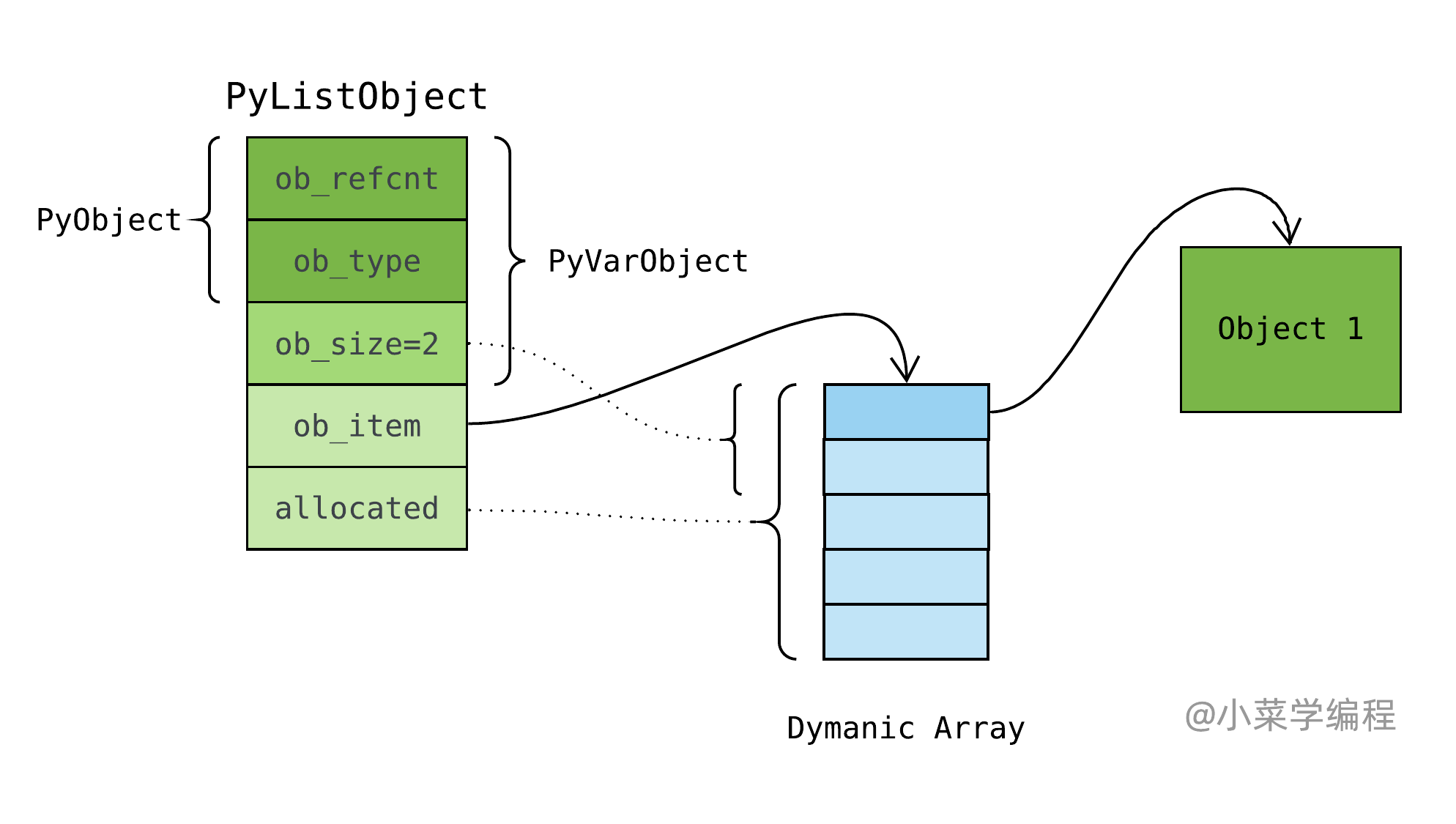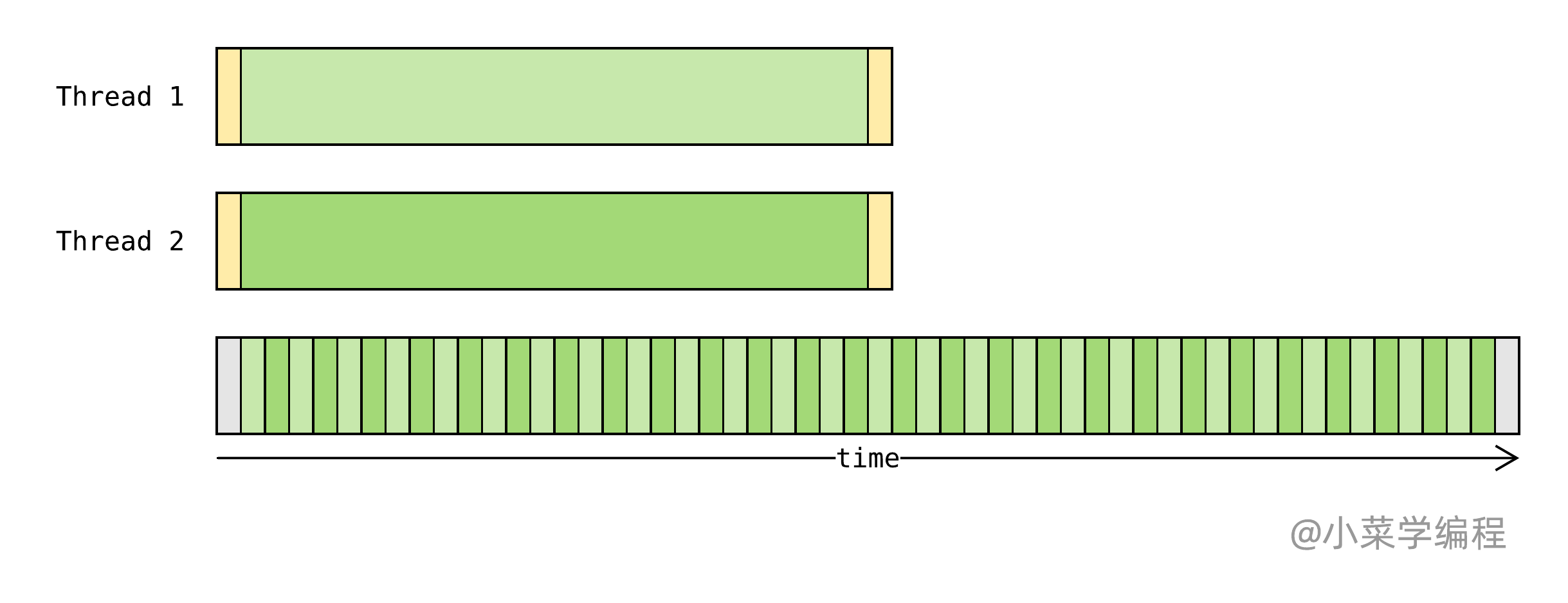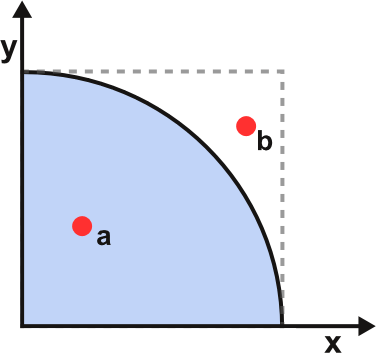# GIL全局锁，束缚Python的紧箍圈

GIL 作为 Python 虚拟机最大的局限性，对 Python 程序运行性能影响深刻。因此，Python 工程师必须明白虚拟机引入 GIL 的前因后果，避免多线程程序被 Python 虚拟机戴上金箍圈，进而产生不可预料的性能问题。

## GIL由来

Python 层面，listdict 等内建对象是线程安全的，这是最基本的常识。研究 listdict 等内建对象源码时，我们并没有看到任何 互斥锁 的痕迹，这多少有点令人意外。以 list 对象 append 方法为例，主要步骤如下：

  1 2 3 4 5 6 7 8 9 10 11 12 13 14 15 16 17 18 19  static int app1(PyListObject *self, PyObject *v) { Py_ssize_t n = PyList_GET_SIZE(self); assert (v != NULL); if (n == PY_SSIZE_T_MAX) { PyErr_SetString(PyExc_OverflowError, "cannot add more objects to list"); return -1; } if (list_resize(self, n+1) < 0) return -1; Py_INCREF(v); PyList_SET_ITEM(self, n, v); return 0; } 
1. 调用 list_resize 将列表长度扩大 1 (第 13-14 行)；
2. 将被追加元素设置到末尾位置(第 16-17 行)；1 2 3 4 5 6 7 8 9 10 11 12  static int some_op(PyListObject *self, ...) { // acquire lock lock(self); // process operation // ... // release lock unlock(self); } 

Python 线程调度实现方式参考了操作系统进程调度中 时间片 的思路，只不过将时间片换成 字节码 。当一个线程取得 GIL 全局锁并开始执行字节码时，对已执行字节码进行计数。IO Blocked 线程一开始阻塞在系统调用上，当系统调用返回后，状态变更为 Ready ，再次等待 GIL 以便获得虚拟机执行权。

GIL 无需频繁加解锁 多进程只能交替执行

## GIL影响

GIL 的束缚下，Python 虚拟机同一时刻只能执行一个线程。这是否意味着多线程完全无法优化程序性能呢？由于程序运行特征千差万别，这个问题得分情况讨论。开始之前，我们先来了解两种不同的运行特征：

1. 可执行 状态，包括 Running 以及 Ready 两种情况，这时竞争处理器资源；
2. 阻塞 状态，一般为等待 IO 处理，这时让出处理器资源；

• 计算密集型 ，程序执行时大部分时间处于 Running 状态；
• IO密集型 ，程序执行时大部分时间处于 IO Blocked 状态；

### IO密集型IO密集型 程序受 GIL 影响相对有限，因为线程在等待 IO 处理时可以让出 GIL 以便其他线程拿到虚拟机执行权：### 计算密集型## 如何提升多核执行能力$π$ 值可以通过随机试验进行估算，具体做法是：制作一个边长为 1 的正方形，绘出内切扇形，如上图。正方形的面积为 1 ，内切扇形的面积为 $\frac{πr^2}{4}$，即 $\frac{π}{4}$。随机往正方形发射一个点，该点落在扇形内的概率为$\frac{π}{4}$。现随机往正方形内发射 $n$ 个点，统计其中落在扇形内的点个数 $in_sectors$，那么 $π$ 值可以这样估算：$π = \frac{4\times in_sectors}{n}$。试验次数越大，估算出来的 $π$ 值也就越准确，当然也意味着巨大的计算量。

  1 2 3 4 5 6 7 8 9 10 11 12  import random def sample(n): in_sectors = 0 # 循环试验n次 for _ in range(n): # 随机生成一个点，坐标均在0到1之间 x, y = random.random(), random.random() # 如果该点到原点的距离平方小于1，则在扇形内 if x*x + y*y < 1: in_sectors += 1 return n, in_sectors 

 1 2  def eval_pi(n, in_sectors): return 4. * in_sectors / n 

 1 2  def estimate_pi(n): return eval_pi(*sample(n)) 

  1 2 3 4 5 6 7 8 9 10 11 12 13 14 15 16 17 18 19 20 21 22 23 24 25 26 27 28 29 30 31 32 33 34 35  from queue import Queue from threading import Thread def estimate_pi_with_threads(n, thread_num): # 用于收集线程执行结果的队列 queue = Queue() # 线程执行函数 def thread_routine(): # 将试验次数n分为thread_num份 # 执行试验并将试验结果放入队列 queue.put(sample(n // thread_num)) # 创建执行线程 threads = [ Thread(target=thread_routine) for _ in range(thread_num) ] # 启动执行线程 for thread in threads: thread.start() # 从任务队列收集试验结果 total_n, total_in_sectors = 0, 0 for thread in threads: n, in_sectors = queue.get() total_n += n total_in_sectors += in_sectors # 回收线程资源 for thread in threads: thread.join() return eval_pi(total_n, total_in_sectors) 

estimate_pi_with_threads 估算函数执行 1 亿次试验耗时 4.63 秒，多线程对程序没有任何提升。

  1 2 3 4 5 6 7 8 9 10 11 12 13 14 15 16 17 18 19 20 21 22 23 24 25 26 27 28 29 30 31 32 33 34  from multiprocessing import Process, Queue as ProcessQueue def estimate_pi_with_processes(n, process_num): # 用于收集子进程执行结果的队列 queue = ProcessQueue() # 子进程执行函数 def process_routine(): # 将试验次数n分为process_num份 # 执行试验并将试验结果放入队列 queue.put(sample(n // process_num)) # 创建执行进程 processes = [ Process(target=process_routine) for _ in range(process_num) ] # 启动执行进程 for process in processes: process.start() # 从任务队列收集试验结果 total_n, total_in_sectors = 0, 0 for process in processes: n, in_sectors = queue.get() total_n += n total_in_sectors += in_sectors # 回收子进程资源 for process in processes: process.join() return eval_pi(total_n, total_in_sectors) 

estimate_pi_with_processes 估算函数执行 1 亿次试验耗时 2.56 秒，几乎比单线程版快了一倍！如果增加子进程数，程序执行速度还可以进一步提升。需要特别注意，超出 CPU 核数的进程数则没有任何意义了。【Python源码剖析】系列文章首发于公众号【小菜学编程】，敬请关注：